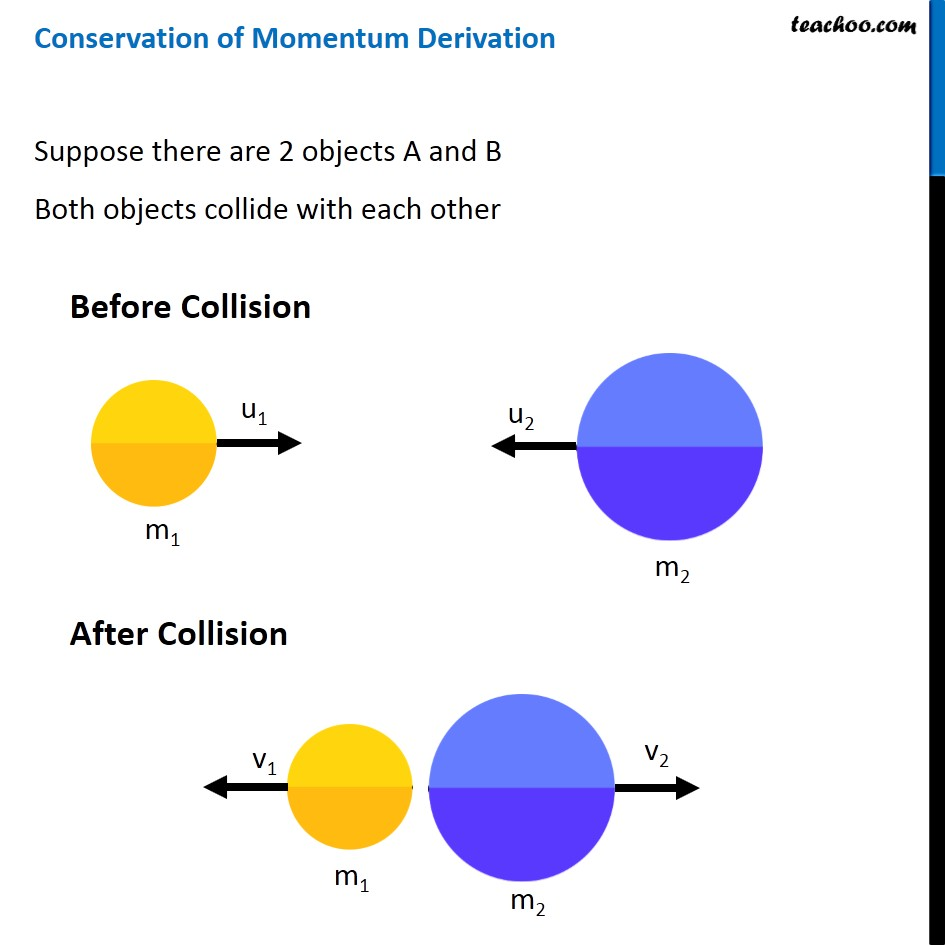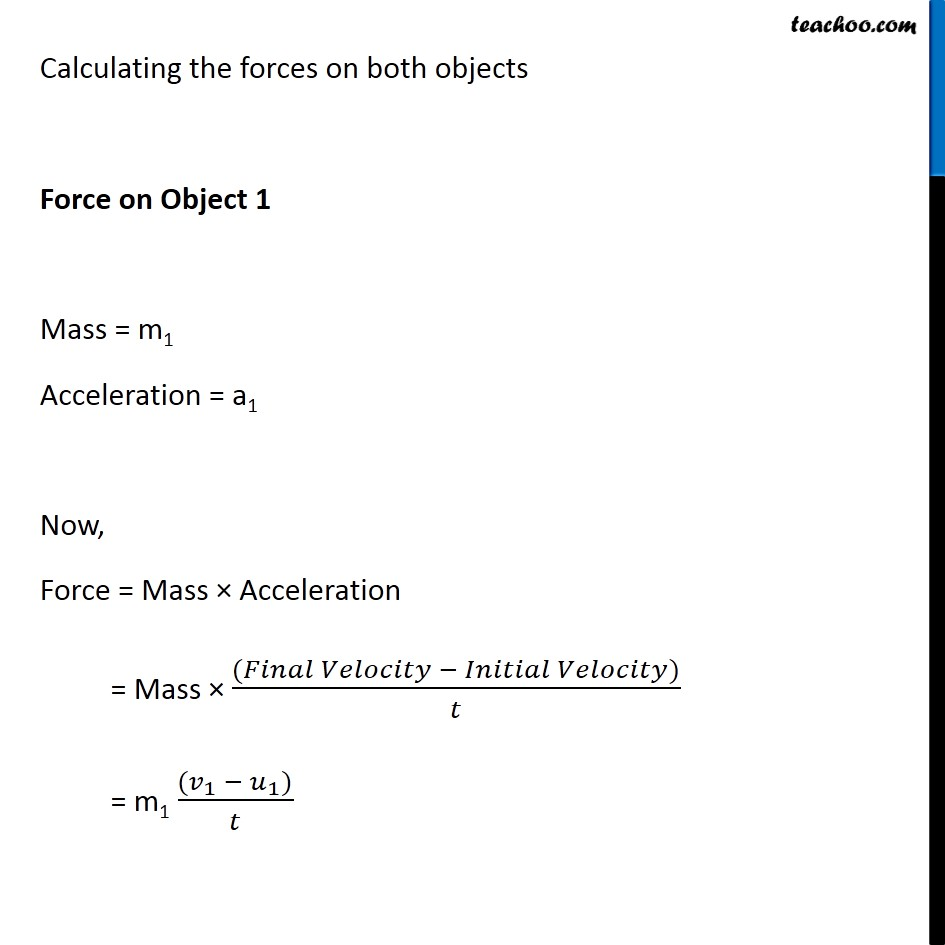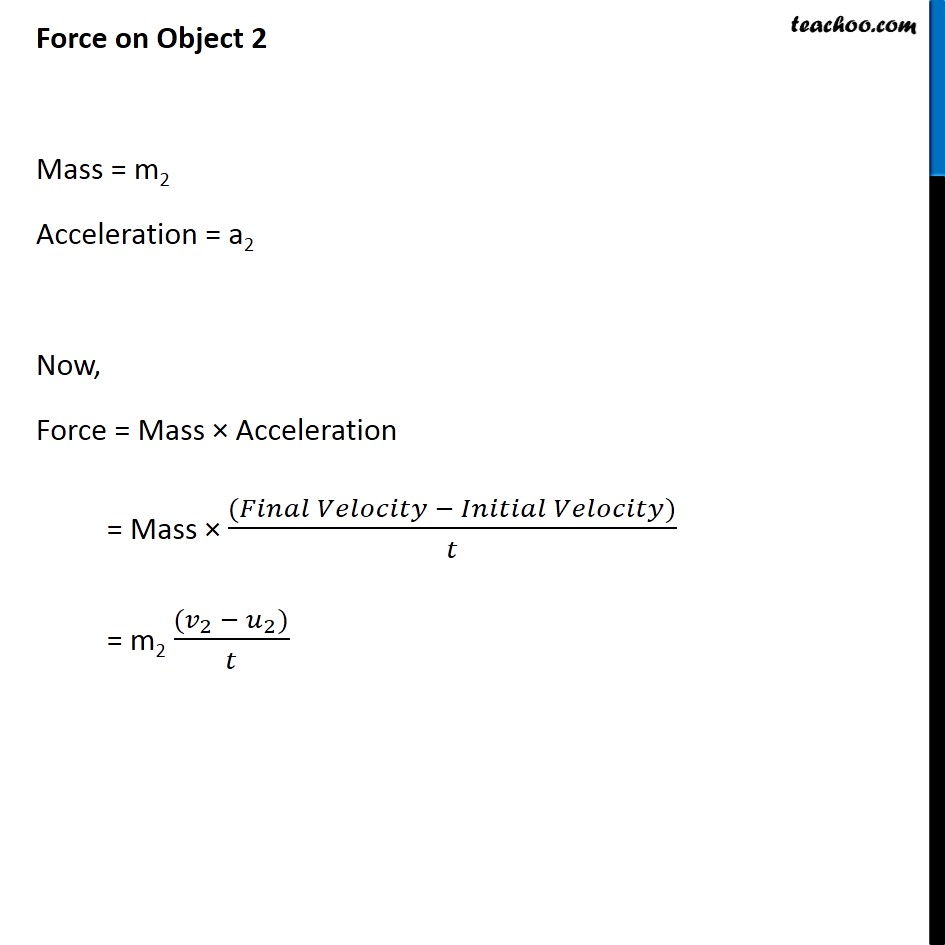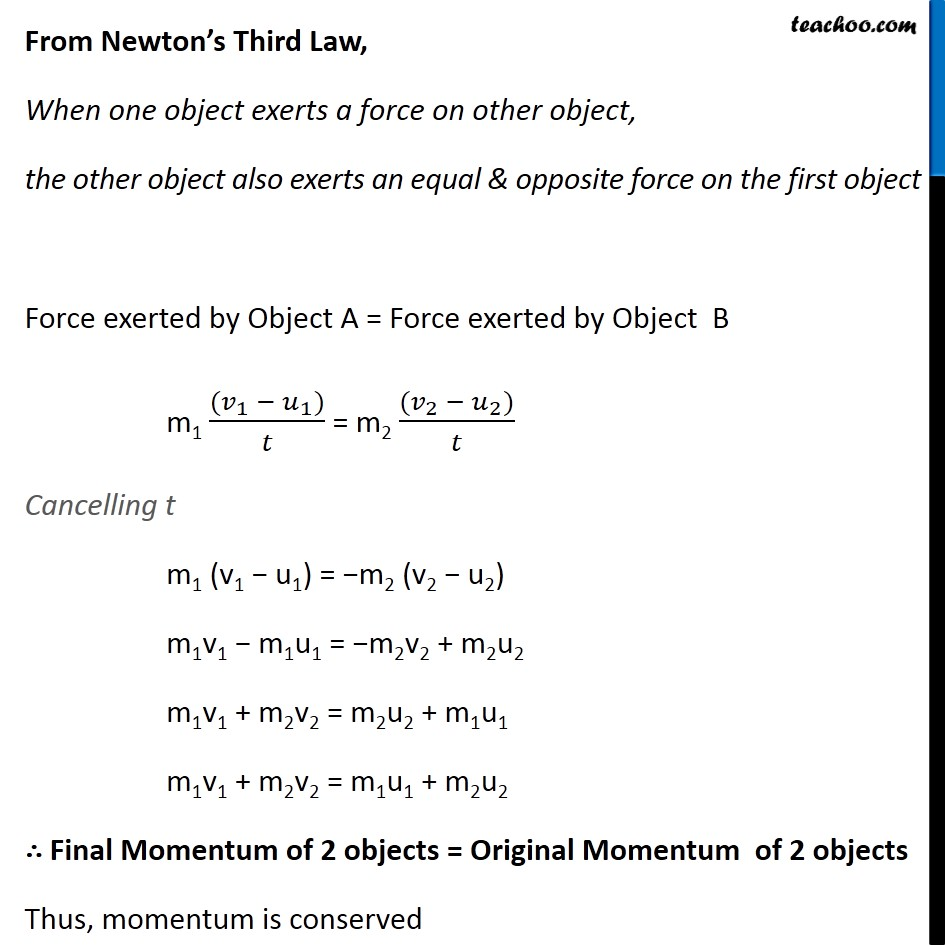Concepts

Class 9
Chapter 9 Class 9 - Force and Laws Of Motion

## According to law of conservation of momentum

When 2 or more bodies act upon one another

Total Momentum remains constant

(provided no other external force is acting)

Hence as per this principle

Momentum before Interaction of 2 bodies = Momentum after Interaction of 2 bodies

Hence as per this principle

Momentum is neither created nor destroyed

It is conserved(remains as it is)## Questions

NCERT Question 11 - Two objects, each of mass 1.5 kg, are moving in the same straight line but in opposite directions. The velocity of each object is 2.5 m s-1 before the collision during which they stick together. What will be the velocity of the combined object after collision?

NCERT Question 15 - An object of mass 1 kg traveling in a straight line with a velocity of 10 m s–1 collides with, and sticks to, a stationary wooden block of mass 5 kg. Then they both move off together in the same straight line. Calculate the total momentum just before the impact and just after the impact. Also, calculate the velocity of the combined object

NCERT Question 17 - Akhtar, Kiran and Rahul were riding in a motorcar that was moving with a high velocity on an expressway when an insect hit the windshield and got stuck on the windscreen. Akhtar and Kiran started pondering over the situation. Kiran suggested that the insect suffered a greater change in momentum as compared to the change in momentum of the motorcar (because the change in the velocity of the insect was much more than that of the motorcar). Akhtar said that since the motorcar was moving with a larger velocity, it exerted a larger force on the insect. And as a result the insect died. Rahul while putting an entirely new explanation said that both the motorcar and the insect experienced the same force and a change in their momentum. Comment on these suggestions.

### Transcript

Conservation of Momentum Derivation Suppose there are 2 objects A and B Both objects collide with each other Before Collision After Collision Calculating the forces on both objects Force on Object 1 Mass = m1 Acceleration = a1 Now, Force = Mass × Acceleration = Mass × ((𝐹𝑖𝑛𝑎𝑙 𝑉𝑒𝑙𝑜𝑐𝑖𝑡𝑦 − 𝐼𝑛𝑖𝑡𝑖𝑎𝑙 𝑉𝑒𝑙𝑜𝑐𝑖𝑡𝑦))/𝑡 = m1 ((𝑣_1 − 𝑢_1))/𝑡 Force on Object 2 Mass = m2 Acceleration = a2 Now, Force = Mass × Acceleration = Mass × ((𝐹𝑖𝑛𝑎𝑙 𝑉𝑒𝑙𝑜𝑐𝑖𝑡𝑦 − 𝐼𝑛𝑖𝑡𝑖𝑎𝑙 𝑉𝑒𝑙𝑜𝑐𝑖𝑡𝑦))/𝑡 = m2 ((𝑣_2 − 𝑢_2))/𝑡 From Newton’s Third Law, When one object exerts a force on other object, the other object also exerts an equal & opposite force on the first object Force exerted by Object A = Force exerted by Object B m1 ((𝑣_1 − 𝑢_1))/𝑡 = m2 ((𝑣_2 − 𝑢_2))/𝑡 Cancelling t m1 (v1 − u1) = −m2 (v2 − u2) m1v1 − m1u1 = −m2v2 + m2u2 m1v1 + m2v2 = m2u2 + m1u1 m1v1 + m2v2 = m1u1 + m2u2 ∴ Final Momentum of 2 objects = Original Momentum of 2 objects Thus, momentum is conserved